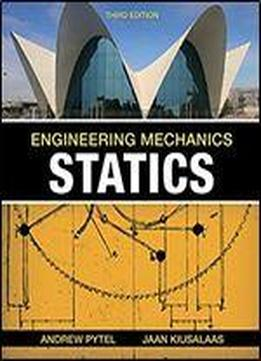# Engineering Mechanics: Statics by Andrew Pytel / 2009 / English / PDF

Now fully incorporated with SI units, these books teach students the basic mechanical behaviour of materials at rest (statics) and in motion (dynamics) while developing their mastery of engineering methods of analysing and solving problems. Traditionally, books for the statics and dynamics courses require students simply to plug problem data into standardised mathematical formulas and then compute an answer without thinking through the problem beforehand. Pytel and Kiusalaas reject this 'plug-and-chug' approach. In sample problems throughout the book, the authors direct students to identify the number of unknowns and independent equations in the problem before they attempt to calculate an answer. In this way, Pytel and Kiusalaas continually train students to think about how and why problems can be solved, by recognising up front whether a problem is statically determinate, or statically indeterminate. Pytel and Kiusalaas is the only textbook that continually reinforces students' ability to recognise determinacy and indeterminacy. Developing this ability in students is a priority for all instructors, especially in the statics course.

views: 441